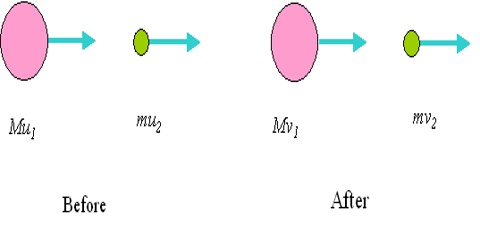# Newton’s Third Law of Motion and Conservation of Momentum

Newton’s Third Law of Motion and Conservation of Momentum

Newton’s third law is nothing but action and reaction. When a body exerts a force on another body, then the second body also exerts the equal and oppositely directed force on the first body. The force that the first body exerts on the second body is considered an action and the force applied by the second body on the first body is called reaction.

Whether the two bodies are at rest or in motion, or one touches the other or not or stay apart from each other Newton’s third law applies in all cases.

The relation of action and reaction is not a causal relation. Action and reaction do not act one after another. Two forces act simultaneously. As long an action acts reaction force also acts for the same duration. When action stops reaction also stops.

In nature force, always act in couples. There is nothing called single isolated force. When we say that one force is active, actually we say one force instead of two active forces. These two forces are complementary to one another.

From the above discussion, we get an idea about Newton’s third law of motion. The law is: To even action there is an equal and opposite reaction i.e., for every action force there is an equal and opposite reacting force. This law may be called the mutual action of forces between the bodies. So, if the action force is F and the reaction force is R, then F = – R.

Explanation: According to Newton’s third law if a body A applies force on another body B, then the body B will also exert equal and opposite force on A. (Figure)Force applied by A is action and force applied B is a reaction. So, if action is F and reaction is R, then F = – R.

Action and reaction act on two different bodies. If there is no action, there will be no reaction. If the duration of action and reaction is t, then,

F x t = R x t

This means, the impulse of the force of action = – impulse of the force of reaction.

It is equally applicable to bodies at rest or in motion.

Newton’s third law of motion and principle of conservation of momentum are explained some examples below;

Books on a table: If a book is kept on a table, weight of the book will exert pressure perpendicularly on the table. It is action. According to Newton’s third law of motion, the table will also exert the upward force on the book. It is a reaction. Since action and reaction are equal and opposite, so the book will remain in stable position on the table.

To fire from a gun: When a hunter fires a shot from the gun, he feels a backward thrust. Initially, the velocity of both the gun and the bullet is zero. As a result, their combined momentum remains zero. When a bullet is fired, it acquires momentum in the forward direction. According to Newton’s third law of motion the gun also acquires an equal and opposite momentum, i.e., the gun will go backward with the same momentum and the hunter will feel backward thrust.

To jump from a boat: When a passenger jumps from a boat on the bank of a river, then the boat is seen to go backward. The boat goes backward and because of the force applied by the passenger on the boat. According to Newton’s third law, the boat also exerts the equal and oppositely directed force on the passenger. As a result, the passenger reaches the shore.